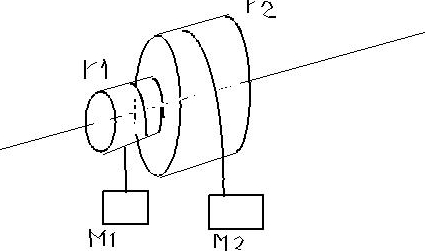# Problem: Two masses m1 and m2 are connected by light cables to the perimeters of two cylinders of radii r1 and r2 respectively, as shown in the diagram above. The cylinders are rigidly connected to each other but are free to rotate without friction on a common axle. The moment of inertia of the pair of cylinders is I = 45kg.m2. Also r1 = 0.5 meter, r2 = 1.5 meter and m1 =20kg.a) Determine m2 such that the system remains in equilibriumThen the mass m2 is removed and the system is released from rest.b) Determine the angular acceleration of the cylindersc) Determine the tension in the cable supporting m1d) Determine the linear speed of m1 at the time it has descended 1 meter.

###### FREE Expert Solution

In this problem, we'll consider the cylinders to be acting as pulleys.

They are counterbalanced by the two masses.
a)

In this part, let's take τ1 to be the torque exerted by m1.

Let's also consider τ2 to be the torque exerted by m2.

The two torques have to balance at equilibrium.

So we'll have:

85% (416 ratings)###### Problem DetailsTwo masses m1 and m2 are connected by light cables to the perimeters of two cylinders of radii r1 and r2 respectively, as shown in the diagram above. The cylinders are rigidly connected to each other but are free to rotate without friction on a common axle. The moment of inertia of the pair of cylinders is I = 45kg.m2. Also r1 = 0.5 meter, r2 = 1.5 meter and m1 =20kg.

a) Determine m2 such that the system remains in equilibrium
Then the mass m2 is removed and the system is released from rest.
b) Determine the angular acceleration of the cylinders
c) Determine the tension in the cable supporting m1
d) Determine the linear speed of m1 at the time it has descended 1 meter.